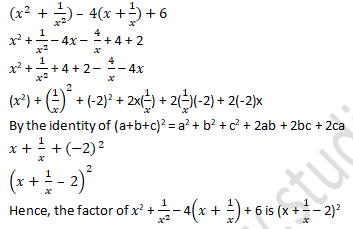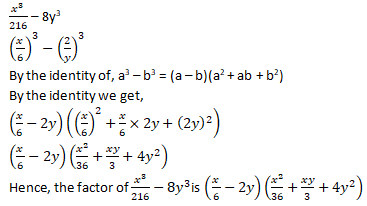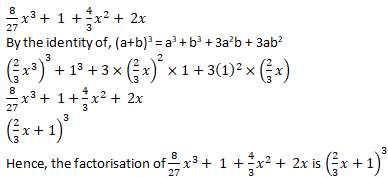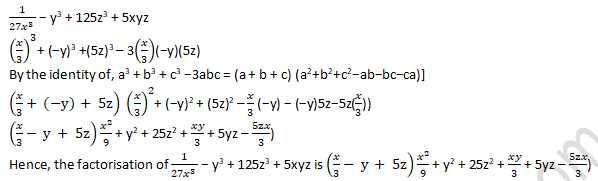# RD Sharma Solutions Class 9 Chapter 5 Factorization of Algebraic Expressions

Read RD Sharma Solutions Class 9 Chapter 5 Factorization of Algebraic Expressions below, students should study RD Sharma class 9 Mathematics available on Studiestoday.com with solved questions and answers. These chapter wise answers for class 9 Mathematics have been prepared by teacher of Grade 9. These RD Sharma class 9 Solutions have been designed as per the latest NCERT syllabus for class 9 and if practiced thoroughly can help you to score good marks in standard 9 Mathematics class tests and examinations

Exercise 5.1
Question 1: Factorize x3 + x – 3x2 – 3
Solution:
It is given that,
x3 + x – 3x2 – 3
The common factor in x3 – 3x2 is x2 and 1 is the common factor in x – 3.
x2 (x – 3) + 1(x – 3)
The common factor in above is (x – 3)
(x – 3) (x2 + 1)
Hence, the factor of x3 + x – 3x2 – 3 is (x – 3) (x2 + 1).

Question 2: Factorize a(a + b)3 – 3a2b(a + b)
Solution:
It is given that,
a(a + b)3 – 3a2b(a + b)
The common factor in a(a + b)3 – 3a2b(a + b) is a(a + b).
a(a + b) [(a + b)2 – 3ab]
a(a + b) [a2 + b2 + 2ab – 3ab]
a(a + b) (a2 + b2 – ab)
Hence, the factor of a(a + b)3 – 3a2b(a + b) is a(a + b) (a2 + b2 – ab).

Question 3: Factorize x(x3 – y3) + 3xy(x – y)
Solution:
It is given that,
x(x3 – y3) + 3xy(x – y)
By using identity of a3 - b3 = (a – b) (a2 + ab + b2)
x(x – y) (x2 + xy + y2) + 3xy(x – y)
The common factor in x(x – y) (x2 + xy + y2) + 3xy(x – y) is x(x - y).
x(x – y) (x2 + xy + y2 + 3y)
Hence, the factor of x(x3 – y3) + 3xy(x – y) is x(x – y) (x2 + xy + y2 + 3y).

Question 4: Factorize a2x2 + (ax2 + 1)x + a
Solution:
It is given that,
a2x2 + (ax2 + 1)x + a
By re-arranging we get,
a2x2 + a + (ax2 + 1)x
Take common in above equation
a(ax2+ 1) + x(ax2 + 1)
(ax2 + 1) (a + x)
Hence, the factor of a2x2 + (ax2 + 1)x + a is (ax2 + 1) (a + x).

Question 5: Factorize x2 + y – xy – x
Solution:
It is given that,
x2 + y – xy – x
By re-arranging we get,
x2 – x – xy + y
Take common in above equation
x(x- 1) – y(x – 1)
(x – 1) (x – y)
Hence, the factor of x2 + y – xy – x is (x – 1) (x – y).

Question 6: Factorize x3 – 2x2y + 3xy2 – 6y3
Solution:
It is given that,
x3 – 2x2y + 3xy2 – 6y3
Take common in above equation
x2(x – 2y) + 3y2(x – 2y)
(x – 2y) (x2 + 3y2)
Hence, the factor of x3 – 2x2y + 3xy2 – 6y3 is (x – 2y) (x2 + 3y2).

Question 7: Factorize 6ab – b2 + 12ac – 2bc
Solution:
It given that,
6ab – b2 + 12ac – 2bc
By re-arranging,
6ab + 12ac – b2 – 2bc (Taking common)
6a(b + 2c) – b(b + 2c)
(b + 2c) (6a – b)
Hence, the factor of 6ab – b2 + 12ac – 2bc is (b + 2c) (6a – b)

Question 8: Factorize (x2  +  1/x2 ) – 4(x+1/x)+6
Solution:
It is given that,Question 9: Factorize x(x – 2) (x – 4) + 4x – 8
Solution:
It is given that,
x(x – 2) (x – 4) + 4x – 8
x(x – 2) (x – 4) + 4(x – 2)
(x – 2) [x(x – 4) + 4]
(x – 2) (x2–4x+4)
(x – 2) [x2–2(x)(2)+ (2)2
By the identity of (a+b)= a2+2ab+b2
(x – 2) (x – 2)
(x – 2)
Hence, the factor of x(x–2) (x–4)+4x–8 is (x – 2)3

Question 10: Factorize (x + 2) (x2 + 25) – 10x2 – 20x
Solution:
It is given that,
(x + 2) (x2 + 25) – 10x2 – 20x
Taking 10 as a common factor,
(x + 2) (x2 + 25) – 10x (x + 2)
By taking common in above equation,
(x + 2) (x2 + 25 – 10x)
(x + 2) (x2 – 10x + 25)
By splitting the middle term we get,
(x+2) (x2 – 5x – 5x + 25)
(x+2) {x (x – 5) – 5 (x – 5)}
(x+2) (x – 5)(x – 5)
(x + 2)(x – 5)2
Hence, the factor of (x + 2) (x2 + 25) – 10x2 – 20x is (x+2) (x– 5)2

Question 11: Factorize 2a2 + 2√6ab + 3b2
Solution:
2a2 + 2√6ab + 3b2
By splitting the middle term we get,
(√2a)2 + 2 × √2a × √3b + (√3b)2
By the identity of (a+b)2 = a2 + b2 + 2ab
a = √2a and b = √3b
(√2 a+√3 b)
Hence, the factor of 2a2 + 2√6ab + 3b2 is (√2a+√3b)2

Question 12:  Factorize (a – b + c)2 + (b – c + a)2 + 2(a – b + c) (b – c + a)
Solution:
It is given that,
(a – b + c)2 + (b – c + a)2 + 2(a – b + c) (b – c + a)
By the identity of (x+y)2 = x2 + y2 + 2xy
Here p = a – b + c and q = b – c + a
[(a – b + c) + (b – c + a)]2
[a – b + c + b – c + a]2
(2a)2
4a2
Hence, the factor of (a – b + c)2 + ( b – c + a)2 + 2(a – b + c) (b – c + a) is 4a2

Question 13: Factorize a2 + b2 + 2(ab + bc + ca)
Solution:
It is given that,
a2 + b2 + 2ab + 2bc + 2ca
By the identity of, (x + y)2 = x2 + y2 + 2xy
= (a+b)2 + 2bc + 2ca
= (a+b)2 + 2c(b + a)
= (a+b)2 + 2c(a + b)…………………………(1)
We can take (a + b) as common factor in the Equation (1)
= (a + b)(a + b + 2c)
Therefore, the factorization of a2 + b2 + 2ab + 2bc + 2ca is (a + b)(a + b + 2c)

Question 14: Factorize 4(x-y)2 – 12(x – y)(x + y) + 9(x + y)2
Solution:
Let us assumed that (x – y) = a, (x + y) = b
= 4a2 – 12ab + 9b2
By splitting the middle term,
= 4a2 – 6ab – 6ab + 9b2
Taking Common 2a and 3b
= 2a(2a – 3b) – 3b(2a – 3b)
= (2a – 3b) (2a – 3b)
= (2a – 3b)2…………………………….(1)
Putting the values back as a = (x – y) , b = (x + y)
= (2a – 3b)2
= [2(x – y) – 3(x + y)]
= [2x – 2y – 3x – 3y]2
= (– x – 5y)2
= (x + 5y)2
Therefore, the factorisation of 4(x - y)2 – 12(x – y)(x + y) + 9(x + y)2 is (x + 5y)2

Question 15: Factorize a2 – b2 + 2bc – c2
Solution:
It is given that,
a2 – b2 + 2bc – c2
By the identity of, (x + y)2 = x2 + y2 + 2xy
= a2 – (b – c)2
By the identity of, x2 - y2 = (x + y)(x - y)
= (a + (b – c)) (a – (b – c))
= (a + b – c) (a – b + c)
Therefore, the factorisation of a2 – b2 + 2bc – c2 is (a + b – c) (a – b + c)

Question 16: Factorize a2 + 2ab + b2 – c2
Solution:
It is given that, a2 + 2ab + b2 – c2
= (a2 + 2ab + b2) – c2
By the identity of, (x + y)2 = x2 + y2 + 2xy
= (a + b)2 – (c)2
By the identity of, x2 - y2 = (x + y)(x - y)
= ((a + b) + c) ((a + b) – c)
Therefore, the factorization of a2 + 2ab + b2 – c2 is (a + b + c) (a + b – c).

Exercise 5.2
Factorize each of the following expressions:
Question 1: p3 + 27
Solution:
It is given that, p3 + 27
We can write it is as,  p3 + 33
By the identity of, (a3 + b3) = (a + b)(a2 –ab + b2)
(p + 3) (p² – 3p – 9)
Hence, the factorisation of p3 + 27 is (p + 3)(p² – 3p – 9).

Question 2: y3 + 125
Solution:
It is given that, y3 + 125
We can write it is as, y3 + 53
By the identity of, (a3 + b3) = (a + b)(a2 –ab + b2)
(y + 5) (y2 − 5y + 52)
(y + 5) (y2 − 5y + 25)
Hence, the factorisation of y3 + 125 is (y + 5) (y2 − 5y + 25)

Question 3: 1 – 27a3
Solution:
It is given that, (1)3 − (3a)3
By the identity of, (a3 + b3) = (a + b)(a2 –ab + b2)
(1 − 3a) (12 + 1×3a + (3a)2)
(1 − 3a) (1 + 3a + 9a2)
Hence, the factorisation of 1 − 27a3 is (1 − 3a)(1 + 3a + 9a2)

Question 4: 8x3y3 + 27a3
Solution:
It is given that, 8x3y3 + 27a3
We can write it as, (2xy)3 + (3a)3
By the identity of, (a3 + b3) = (a + b)(a2 –ab + b2)
(2xy +3a)((2xy)2−2xy×3a+(3a)2)
(2xy+3a)(4x2y2 −6xya + 9a2)
Hence, the factorisation of 8x3y3 + 27a3 is (2xy+3a)(4x2y2 −6xya + 9a2)

Question 5: 64a3 − b3
Solution:
We have,
64a3 − b3
(4a)3 − b3
By the identity of, a3 – b3 = (a – b)(a2 + ab + b2)
(4a−b) ((4a)2 + 4a×b + b2)
(4a−b) (16a2 +4ab+b2)
Hence, the factorisation of 64a3 − b3 is (4a−b) (16a2 + 4ab + b2

Question 6: (x3 )/216 – 8y3
Solution:
We have,Question 7: 10x4y – 10xy4
Solution:
We have,
10x4y – 10xy4
10xy(x3− y3)
By the identity of, a3 – b3 = (a – b)(a2 + ab + b2)
By the identity we get,
10xy (x−y)(x2 + xy + y2)
Thus, the factor of 10x4y – 10xy4 is 10xy (x−y)(x2 + xy + y2)

Question 8: 54x6y + 2x3y4
Solution:
We have,
54x6y + 2x3y4
2x3y(27x3 +y3)
2x3y((3x)3 + y3)
By the identity of, a3 + b3 = (a + b)(a2 – ab + b2)
By the identity we get,
2x3y {(3x+y) ((3x)2−3xy+y2)}
Hence, the factor of 54x6y + 2x3y4 is 2x3y(3x+y)(9x2− 3xy + y2).

Question 9: 32a3 + 108b3
Solution:
We have,
32a3 + 108b3
4(8a3 + 27b3)
4((2a)3+(3b)3)
By the identity of, a3 + b3 = (a + b)(a2 – ab + b2)
By the identity we get,
4[(2a+3b)((2a)2−2a×3b+(3b)2)]
Hence, the factor of 32a3 + 108b3 is 4(2a+3b)(4a2 − 6ab + 9b2)

Question 10: (a−2b)3 − 512b3
Solution:
We have,
(a−2b)3 − 512b3
(a−2b)3 −(8b)3
By the identity of, a3 – b3 = (a – b)(a2 + ab + b2)
By the identity we get,
(a −2b−8b) {(a−2b)2 + (a−2b)8b + (8b)2}
(a −10b)(a2 + 4b2 − 4ab + 8ab − 16b2 + 64b2)
Hence, the factor of (a−2b)3 − 512b3 is (a−10b)(a2 + 52b2 + 4ab)

Question 11: (a+b)3 − 8(a−b)3
Solution:
We have,
(a+b)3 − 8(a−b)3
(a+b)3 − [2(a−b)]3
(a+b)3 − [2a−2b]3
By the identity of, x3 – y3 = (x – y)(x2 + xy + y2)]
By the identity we get,
(a+b−(2a−2b))((a+b)2+(a+b)(2a−2b)+(2a−2b)2)
(a+b−2a+2b)(a2+b2+2ab+(a+b)(2a−2b)+(2a−2b)2)
(a+b−2a+2b)(a2+b2+2ab+2a2−2ab+2ab−2b2+(2a−2b)2)
(3b−a)(3a2+2ab−b2+(2a−2b)2)
(3b−a)(3a2+2ab−b2+4a2+4b2−8ab)
(3b−a)(3a2+4a2−b2+4b2−8ab+2ab)
Hence, the factor of (a+b)3 − 8(a−b)3 is (3b−a)(7a2+3b2−6ab)

Question 12: (x+2)3 + (x−2)3
Solution:
We have,
(x+2)3 + (x−2)3
By the identity of, a3 + b3 = (a + b)(a2 – ab + b2)]
(x+2+x−2)((x+2)2−(x+2)(x−2)+(x−2)2)
2x(x2 +4x+4−(x+2)(x−2)+x2−4x+4)
By the identity of, (a+b)(a−b) = a2 − b
2x(2x2 + 8 − (x2 − 22))
2x(2x2 +8 − x2 + 4)
2x(x2 + 12)
Hence, the factor of (x+2)3 + (x−2)3 is 2x(x2 + 12)

Exercise 5.3
Question 1: Factorize 64a3 + 125b3 + 240a2b + 300ab2
Solution:
We have,
64a3 + 125b3 + 240a2b + 300ab2
By the identity of (a+b)3 = a3 + b3 + 3a2b + 3ab2
(4a)3 + (5b)3 + 3(4a)2(5b) + 3(4a)(5b)
(4a+5b)3
Hence, the factorization of 64a3 + 125b3 + 240a2b + 300ab2 is (4a+5b)3

Question 2: Factorize 125x3 – 27y3 – 225x2y + 135xy2
Solution:
We have,
125x3 – 27y3 – 225x2y + 135xy2
By the identity of,  (a−b)3 = a3 − b3 − 3a2b + 3ab
(5x)3−(3y)3−3(5x)2(3y) + 3(5x)(3y)2
(5x − 3y)3
Hence, the factorization of 125x3 – 27y3 – 225x2y + 135xy2 is (5x − 3y)3

Question 3: Factorize 8/27 x3+ 1 +4/3 x2+ 2x
Solution:
We have,Question 4: Factorize 8x3 + 27y3 + 36x2y + 54xy2
Solution:
We have,
8x3 + 27y3 + 36x2y + 54xy2
By the identity of, (a+b)3 = a3 + b3 + 3a2b + 3ab2
(2x)3 + (3y)3 + 3×(2x)2×3y + 3×(2x)(3y)2
(2x+3y)3
Hence, the factorisation of 8x3 + 27y3 + 36x2y + 54xy2 is (2x+3y)3

Question 5: Factorize a3 − 3a2b + 3ab2 − b3 + 8
Solution:
We have,
a3 − 3a2b + 3ab2 − b3 + 8
By the identity of,  (a−b)3 = a3 − b3 − 3a2b + 3ab2
(a−b)3 + 23
By the identity of, a3 + b3 =(a + b)(a2 – ab + b2)]
(a−b+2)((a−b)2−(a−b) × 2 + 22)
(a−b+2)(a2+b2−2ab−2(a−b)+4)
(a−b+2)(a2+b2−2ab−2a+2b+4)
Hence, the factorisation of a3 − 3a2b + 3ab2 − b3 + 8 is (a−b+2)(a2+b2−2ab−2a+2b+4)

Exercise 5.4
Factorize each of the following expressions:

Question 1:  a3 + 8b3 + 64c3 − 24abc
Solution:
We have,
a3 + 8b3 + 64c3 − 24abc
(a)3 + (2b)3 + (4c)3− 3× a × 2b × 4c
By the identity of, a3+b3+c3−3abc = (a + b + c) (a2+b2+c2−ab−bc−ca)
(a+2b+4c) (a2+(2b)2 + (4c)2−a× 2b − 2b × 4c − 4c × a)
(a+2b+4c) (a2 +4b2 +16c2 −2ab−8bc−4ac)
Therefore, the factorisation of a3 + 8b3 + 64c3 − 24abc is (a+2b+4c) (a2 +4b2 +16c2 −2ab−8bc−4ac)

Question 2: x3 − 8y3+ 27z3 + 18xyz
Solution:
We have,
x3 − 8y3+ 27z3 + 18xyz
x3 − (2y)3 + (3z)3 – 3×x× (−2y)(3z)
(x + (−2y) + 3z) (x2 + (−2y)2 + (3z)2 −x(−2y) − (−2y)(3z) −3z(x))
By the identity of, a3 + b3 + c3 − 3abc = (a + b + c) (a2 + b2 + c2 – ab – bc − ca)
(x −2y + 3z) (x2 + 4y2 + 9z2 + 2xy + 6yz − 3zx)
Hence, the factorisation of x3 − 8y3+ 27z3 + 18xyz is (x −2y + 3z) (x2 + 4y2 + 9z2 + 2xy + 6yz − 3zx)

Question 3: 27x3 − y3– z3 – 9xyz
Solution:
We have,
27x3 − y3– z3 – 9xyz
(3x)3 − y3– z3 – 3(3xyz)
By the identity of, a3 + b3 + c3 −3abc = (a + b + c)(a2+b2+c2−ab−bc−ca)]
(3x – y – z) { (3x)2 + (- y)2 + (– z)2 + 3xy – yz + 3xz)}
(3x – y – z) { 9x2 + y2 + z2 + 3xy – yz + 3xz)}
Hence, the factorisation of 27x3 − y3– z3 – 9xyz is (3x – y – z) {9x2 + y2 + z2 + 3xy – yz + 3xz)}

Question 4:  1/(27x3) − y3 + 125z3 + 5xyz
Solution:
We haveQuestion 5: 8x3 + 27y3 − 216z3 + 108xyz
Solution:
We have,
8x3 + 27y3 − 216z3 + 108xyz
(2x)3 + (3y)3 +(−6y)3 −3(2x)(3y)(−6z)
By the identity of, a3 + b3 + c3 −3abc = (a + b + c)(a2+b2+c2−ab−bc−ca)]
(2x + 3y + (−6z)) {(2x)2 + (3y)2 + (−6z)2 −2x × 3y − 3y × (−6z) − (−6z) × 2x }
(2x +3y − 6z) {4x 2 +9y2 +36z2 −6xy + 18yz + 12zx}
Hence, the factorisation of 8x3 + 27y3 − 216z3 + 108xyz is (2x +3y − 6z) {4x 2 +9y2 +36z2 −6xy + 18yz + 12zx}

Question 6: 125 + 8x3 − 27y3 + 90xy
Solution:
We have,
125 + 8x3 − 27y3 + 90xy
(5)3 + (2x)3 +(−3y)3 −3 × 5 × 2x ×(−3y)
By the identity of, a3 + b3 + c3 −3abc = (a + b + c)(a2+b2+c2−ab−bc−ca)]
(5 + 2x + (−3y)) (52 +(2x)2 +(−3y)2 −5(2x)−2x(−3y) − (−3y)5)
(5 + 2x − 3y)(25 + 4x2 +9y2 − 10x + 6xy + 15y)
Hence, the factorisation of 125 + 8x3 − 27y3 + 90xy is (5 + 2x − 3y)(25 + 4x2 +9y2 − 10x + 6xy + 15y)

Question 7: (3x−2y)3 + (2y−4z)3 + (4z−3x)3
Solution:
We have,
(3x−2y)3 + (2y−4z)3 + (4z−3x)3
Let us assumed that,
a = (3x−2y)
b = (2y−4z)
c = (4z−3x)
By the identity of, a3 + b3 + c3 −3abc = (a + b + c)(a2+b2+c2−ab−bc−ca)]
a + b + c = (3x−2y) + (2y−4z) + (4z−3x)
a + b + c = 3x−2y + 2y − 4z + 4z − 3x
a + b + c = 0
a3 + b3 + c3 − 3abc = 0 (a2+b2+c2−ab−bc−ca)]
a3 + b3 + c3 = 3abc
Put the value of a, b and c in the equation (1)
(3x−2y)3 + (2y−4z)3 + (4z−3x)3 = 3(3x−2y)(2y−4z)(4z−3x)
Hence the value of (3x−2y)3 + (2y−4z)3 + (4z−3x)3 is 3(3x−2y)(2y−4z)(4z−3x).

Question 8: (2x−3y)3 + (4z−2x)3 + (3y−4z)3
Solution:
We have,
(2x−3y)3 + (4z−2x)3 + (3y−4z)3Let us assumed that
a = (2x – 3y)
b = (4z – 2x)
c = (3y – 4z)
By the identity of, a3 + b3 + c3 −3abc = (a + b + c)(a2+b2+c2−ab−bc−ca)]
a + b + c = (2x – 3y) + (4z – 2x) + (3y – 4z)
a + b + c = 2x – 3y + 4z – 2x + 3y – 4z
a + b + c = 0
a3 + b3 + c3 − 3abc = 0 (a2+b2+c2−ab−bc−ca)]
a3 + b3 + c3 = 3abc
(2x−3y)3 + (4z−2x)3 + (3y−4z)3 = 3(2x−3y)(4z−2x)(3y−4z)
Hence the value of (2x−3y)3+(4z−2x)3 +(3y−4z)3 is (2x−3y)3 + (4z−2x)3 +(3y−4z)3 = 3(2x−3y)(4z−2x) (3y−4z)

Exercise Very Short Answer Questions :->

Question 1: Factorize x4 + x2 + 25
Solution:
We have,
x4 + x2 + 25
(x2)2 + 52 + x2
By the identity of, a2 + b2 = (a + b)2 – 2ab
(x2 + 5)2 −2(x2) (5) + x2
(x2 + 5)2 −10x2 + x2
(x2 + 5)2 − 9x2
(x2 + 5)2 − (3x)2
By the identity of, a2 – b2 = (a + b) (a – b)
(x2 + 5 + 3x) (x2  + 5 − 3x)
Hence, the factorisation of x4 + x2 + 25 is (x2 + 5 + 3x) (x2  + 5 − 3x).

Question 2: Factorize x2 – 1 – 2a – a2
Solution:
We have,
x2 – 1+2a+a2
x2 – ((1)2+2a+a2
By the identity of, (a + b)2 = a2 + b2 + 2ab
x2 – (a + 1)2
By the identity of, a2 – b2 = (a + b) (a – b)
(x + (a + 1) (x - (a + 1)
(x + a + 1) (x - a - 1)
Hence, the factorisation of x2 – 1 – 2a – a2 is (x + a + 1) (x - a - 1)

Question 3: If a + b + c = 0, then write the value of a3 + b3 + c3.
Solution:
It is given that, a + b + c =0
By the identity of, a3 + b3 + c3 −3abc = (a + b + c)(a2+b2+c2−ab−bc−ca)]
a3 + b3 + c3 – 3abc = (a + b +c) (a2 + b2 + c2 – ab – bc − ca)
a3 + b3 + c3 – 3abc = 0 (a2 + b2 + c2 – ab – bc − ca)
a3 + b3 + c3 – 3abc = 0
a3 + b3 + c3 = 3abc
Hence, the value of a3 + b3 + c3 is 3abc.

Question 4: If a2 + b2 + c2= 20 and a + b + c = 0, find ab + bc + ca.
Solution:
It is given that, a2 + b2 + c2 = 20 and a + b + c = 0
By the identity of, (a+b+c)² = a² + b² + c² + 2(ab + bc + ca)
(a+b+c)² = a² + b² + c² + 2(ab + bc + ca)…………………………..(1)
Put the value of a2 + b2 + c2 and a + b + c in equation (1)
(0)² = a² + b² + c² + 2(ab + bc + ca)
0 = 20 + 2(ab + bc + ca)
-20 = 2(ab + bc + ca)
-10 = ab + bc + ca
ab + bc + ca = -10
Hence, the value of ab + bc + ca is ab + bc + ca = -10

Question 5: If a + b + c = 9 and ab + bc + ca = 40, find a2 + b2 + c2
Solution:
It is given that,
a + b + c = 9
ab + bc + ca = 40
By the identity of, (a+b+c)² = a² + b² + c² + 2(ab + bc + ca)
(a+b+c)² = a² + b² + c² + 2(ab + bc + ca)……………..(i)
Put the value of a + b + c and ab + bc + ca in equation (1)
(9)2 = a² + b² + c² + 2(40)
81 = a² + b² + c² + 80
81 – 80 = a² + b² + c²
1 = a² + b² + c²
a² + b² + c² = 1
Hence, the value of a² + b² + c² is 1.